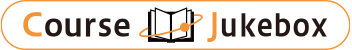### CourseDetailDegree
Bachelor
3
Course delivery methods
face-to-face
Subject
Physical sciences, Mathematical sciences, Engineering & technology
Program
School
School of Science and Engineering
Department
Bachelor's Program in Interdisciplinary Engineering
Campus
Tsukuba Campus
Classroom
3A408
Course Offering Year
2023-2024
Course Offering Month
January - February
Weekday and Period
Wed4,5
Capacity
Credits
1.0
Language
English
Course Number
FJ12111

### Statistical Physics II University of Tsukuba

#### Course Overview

The fundamental concepts introduced in Statistical Physics I are applied to a few simple physical systems such as ideal gases. We derive the classical (Boltzmann) and quantum (Fermi-Dirac and Bose-Einstein) statistics from statistical ensembles. The fundamental principles underlying when extracting the maximum work from heat are clarified. Those principles are applied to simple systems such as (classical and quantum) ideal gas and conduction electrons in metals.

#### Learning Achievement

1. The students are able to construct the micro-canonical, canonical, grand- canonical ensembles and the corresponding statistical probabilities.
2. The students are able to derive the fundamental thermodynamic functions such as entropy, Helmholtz free energy, and thermodynamic potential from the statistical ensembles.
3. The students are able to calculate the pressure of an ideal gas from the Maxwell velocity distribution.

#### Competence

Related to 2. Understanding ability of physical phenomena.

#### Course prerequisites

Statistical Mechanics I
Intro. to Single-Variable Calculus I & II, Advanced Calculus, Linear Algebra I & II, Probability and Statistics, Mechanics, Electromagnetism, Thermodynamics, Modern Physics

Homework & Class performance 30 %
Final 70%

#### Course schedule

Fundamental equation and Gibbs-Duhem relation
Legendre transformation
Other fundamental equations
Properties of ideal gas
Thermodynamic coefficients
van der Waals gas
Canonical ensemble
Partition function and Helmholtz free energy
Grand-canonical ensemble
T-p distribution and Gibbs free energy

Lectures